## Trigonometry Simplify – sin^4x – cos^4x

I thought I’d post this quick simplification using trig identities since it gave me a bit of a run for my money.  Partially so I remember it better and second, to lend a bit of help to those who might be stuck.

The Problem: Simplify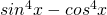Now, how hard could this be?!?  As it turns out, it’s not too bad, but the trick is in factoring what we have.

Factor things out: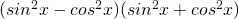Great!  Now that we have this in better form we need to bring in a couple of trig identities which will help us solve this and one that I’m going to cite because it could trick you!

Pythagorean Identity 1:Pythagorean Identity 2: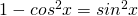Pythagorean Identity 3: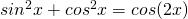(This is the tricky one!  As much as it seems like we could use this identity we would end up loosing terms.  Don’t use this)

Now, things should start looking a bit simpler to solve.  So, here we go starting with what we factored:

1)2) Use Identity 1 to substitute for the first term: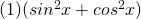3) Use Identity 2 to substitute for the first part of the second term:4) Now combine the two like terms: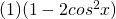5) And so we have it!  The final result is: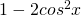Now, looking back, if you notice in identity 3, if you use it, you end up with a term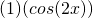which multiplied out you loose the negative and the 2 in front of cos.  This is a big problem because if you did it this way, what you get in the end does not equal what you had in the beginning and thus, isn’t a valid way to simplify this problem.  As a result, you must use identities 1 and 2 as shown in the 5 steps above.

Cheers!

## Related Rates, Snowball

I’m writing this article because I felt that it was significant to understanding a good deal of physics and astronomy aspects that rely on this type of mathematics.  By the very title, I’ve implied the need to apply the big bad demon known as Calculus.  Had I better understood this part of calculus sooner it would have made a significant difference in my ability to handle some of the more complex physics problems.  As a result, I thought I would share in hopes that this might help someone in the future.

Although this article will focus on the shortcut methods to working out some of the problems presented and the need for in-depth understanding of calculus is not needed.  To make sense of everything the reader should have a handle on basic geometry and trigonometry as well as some familiarity with the following calculus topics:

• Functions
• Continuity and Limits
• Differentials
• Partial Differential Equations

So, let’s begin with the classic melting snowball problem:

A spherical snowball is melting in such a way that its radius is decreasing at rate of 0.5 cm/min. At what rate is the volume of the snowball decreasing when the radius is 3 cm. (Note the answer is a positive number).

Step 1:  Identify what we have and what we’re looking for.

Radius: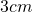Decreasing Rate: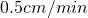decreasing radius
Additional Information:  We also have a sphere and we know that the sphere is decreasing which means that it’s volume is also decreasing.  Thus, we need the formula for the volume of a sphere since that’s what’s changing: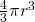Step 2:  Now for the trick.  One very handy piece of calculus that allows us to handle related rates problems is differentiation, specifically the differential.  It is important to note that this is not exactly the same thing as taking the derivative, but that action is one process in this problem.  The resulting formula for this process is: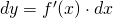Step 3:  To break this formula down a bit, dy (since we’re working with volume this will be dV for us) is the part you’re looking for in this problem; solving for it will give you the rate at which the volume is changing in relation to the radius of the sphere.  The next part, f'(x) is the derivative of the formula that you’re working with, in this case, the formula for the area of a sphere.  Finally, dx is the current value of the rate of change (or slope of the tangent line to the function at the given point, if that makes more sense) of whatever it is we happen to be looking for.  In this case, it would be the rate that the radius of the sphere is changing.

Step 4:  Now that we’ve established our variables and the equation needed to solve this problem we can set up the problem further.

We must find the derivative of the equation of the volume of a sphere: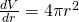Now, substitute in the values into our differential: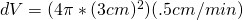Now solve: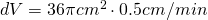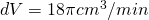or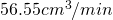And there we have it; when the radius of the snowball is 3 centimeters, the volume of the  snowball is decreasing by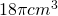or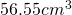every minute!  It must be one hot day out!